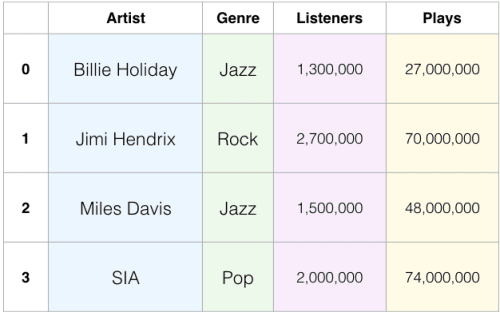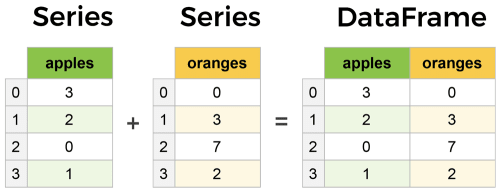# Learning Data Analysis with Python - Introduction to Pandas

## What is Pandas and Why do we need it

Pandas is python library that provides rich data structures and functions that makes working with relational and structured data easy, fast and convenient. It is developed on top of the Numpy package for the high performance computing and it gives flexible data manipulation techniques of relational databases. Okay, now why do we need panadas at all? If we want to mainpulate high dimensional data, we have numpy, so why do we need it? Well, indeed numpy is there for the high dimensional data manipulation but what it doesn't provide is a way to index or label our data like this.And, although we can do so many interesting things to our data using numpy functions such as finding statistics like mean,median,mode and standard deviation but we can't fill missing values in our data. Numpy doesn't provide any built-in method to handle missing values in our data and in real world problems, we don't always come around the perfect datasets.

There are other limitations to numpy such as no way to group data and no way to pivot data, and thus, Pandas was created to help programmers and data scientists with the data analysis task in Python.

## Data Structures in Pandas

Well as we've mentioned earlier, pandas provides rich data structures and functions, let's start by looking at the data structures and then we'll look at the functions and methods that we can apply on these data structures to crunch data.

Pandas provide two major data structures namely "Series" and "DataFrames". In plain english, Series is a one-dimensional labeled array, whereas DataFrames is two-dimensional labeled array with columns of potentially different types. Although, Series are useful in many tasks, DataFrames are the most commonly used pandas object. You can think of DataFrame as an SQL table.

Let's look at the example for both of these data structures.Let's start by looking at the different ways of creating a Series object.But, Before we get into coding, we need to install pandas as python package. This can be done using `pip install pandas` or you can install it using `conda install pandas` if you are using Anaconda Distribution for Python. If you don't have python installed on your machine, the best way to get started without all the hustle of installing it is Google Colab. Learn more about it here. I'm going to use jupyter notebooks which you can find here.

## Series

As mentioned above, Series is a one-dimensional array-like object containing an array of data and an array of labels associated with the data. The data labels are called 'index'. We can create a Series from a list or a dictionary or a numpy array. Let's start with a list.

## Series using a list

``````In:
import pandas as pd
import numpy as np

friends = ["Monica", "Chandler", "Joey", "Pheobe", "Ross", "Rachel"]
series = pd.Series(friends)
series

Out:
0      Monica
1    Chandler
2        Joey
3      Pheobe
4        Ross
5      Rachel
dtype: object``````

As we can see, we get the Series object with the data that we provided with the associated index of for each row. We can access our data from this `series` using the index just like we do with the list.

``````In:
series

Out:
'Monica'``````

Or we can get all the values in a list using `.value` attribute on series object.

``````In:
series.values

Out:
array(['Monica', 'Chandler', 'Joey', 'Pheobe', 'Ross', 'Rachel'],
dtype=object)``````

Similarly, we can get all the indices in a list using `.index` attribute.

``````In:
series.index

Out:
RangeIndex(start=0, stop=6, step=1)``````

Note something different? Right, it doesn't return a list of indices as it did in values, that's because the implicit index that pandas automatically creates for us is just a list of consecutive numbers starting from 0. A more intiuative approach would be to provide index identifying each data point while creating a Series.

``````In:
nick_names = ["Monana", "Officer Bing", "Ken Adams", "Regina Filange", "Dinosaur Guy", "Fun Aunt Rachel"]
series = pd.Series(data = friends, index = nick_names)
series

Out:
Monana               Monica
Officer Bing       Chandler
Regina Filange       Pheobe
Dinosaur Guy           Ross
Fun Aunt Rachel      Rachel
dtype: object``````

And now, we can access our data using the index that we provided like this.

``````In:
series["Dinosaur Guy"]

Out:
'Ross'``````

It's clearly a bad example of indexing but you got the idea, right?

## Series using a dictionary

Now, let's instantiate Series from a dictionary. When creating a Series from dictionaries, we don't pass index, the Series gets ordered by dictionary's insertion order and the keys of dictionary becomes indices of Series.

``````In:
d = {"a" : 1, "b" : 2, "c" : 3}
series = pd.Series(d)
series

Out:
a    1
b    2
c    3
dtype: int64``````

Series object also works like dictionaries. You can get or set the values in the Series by index label just like dictionaries.

``````In:
series["c"]

Out:
3

In:
series["d"] = 4
series

Out:
a    1
b    2
c    3
d    4
dtype: int64``````

## Series using a numpy array

And finally, we can create a Series from a numpy array.

``````In:
d = np.random.randn(5) # Create a numpy array of 5 random numbers
series = pd.Series(d)
series

Out:
0   -0.507243
1   -0.115731
2    0.526216
3   -0.495840
4    0.065357
dtype: float64``````

Series is also similar to numpy array in functionalities. We can index data from a Series object as we do from a numpy array.

``````In
series[:3] # Returns first 3 elements

Out:
0   -0.507243
1   -0.115731
2    0.526216
dtype: float64

In:
series[series > 0] # Returns elements whose value is greater than 0

Out:
2    0.526216
4    0.065357
dtype: float64``````

Now, once we created a Series, we can apply many different methods on it to achieve different tasks. A few of them are as below. Read more about all the methods available on Series object at pandas official documentation.

``````In:
series.size # Returns size of the series.
Out:
5

In:
series.shape # Returns shape of the series.
Out:
(5,)

In:
series.add(1) # Returns series with elements' value increased by 1
Out:
0    0.492757
1    0.884269
2    1.526216
3    0.504160
4    1.065357
dtype: float64

In:
series.sub(1) # Returns series with elements' value decreased by 1
Out:
0   -1.507243
1   -1.115731
2   -0.473784
3   -1.495840
4   -0.934643
dtype: float64

In:
series.mul(10) # Returns series with elements' value multiplied by 10
Out:
0   -5.072431
1   -1.157307
2    5.262160
3   -4.958401
4    0.653565
dtype: float64

In:
series.div(10) # Returns series with elements' value divided by 10
Out:
0   -0.050724
1   -0.011573
2    0.052622
3   -0.049584
4    0.006536
dtype: float64

In:
series.abs() # Returns series with absolute value of elements
Out:
0    0.507243
1    0.115731
2    0.526216
3    0.495840
4    0.065357
dtype: float64

In:
series.max() # Returns maximum of all the elements.
Out:
0.5262159987471329

In:
series.min() # Returns minimum of all the elements.
Out:
-0.5072430551642492

In:
series.sum() # Returns sum of all the elements.
Out:
-0.5272413324692681

In:
series.mean() # Returns mean of the series.
Out:
-0.10544826649385361

In:
series.median() # Returns median of the series.
Out:
-0.1157306666767307

In:
series.std() # Returns standard deviation of the series.
Out:
0.43073327047221427

In:
series.drop(4) # Returns series with specified index removed.
Out:
0   -0.507243
1   -0.115731
2    0.526216
3   -0.495840
dtype: float64

In:
series
Out:
0   -0.507243
1   -0.115731
2    0.526216
3   -0.495840
4    0.065357
dtype: float64``````

Look at the last code when we print the original series object, we get the elements same as we created, because all the operations that we applied on the series object gives us the view of the series and doesn't affect the original object. Okay, so this is just a beginning, there are many more interesting things that we can do with pandas such as dropping null values or replacing them with some other values from data, visualize data using so many plots and charts, combine and merge two or more Series, create and manipulate Time Series, etc.

That's it for this post.In the next couple of posts, I'll be playing with some real world datasets and make some analysis on those data using Pandas.

Get full code notebook here : Github